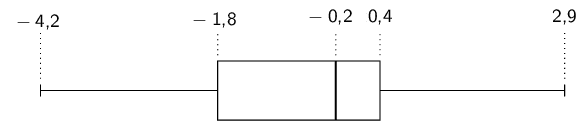Home Practice
For learners and parents For teachers and schools
Textbooks
Full catalogue
Pricing SupportLog in

We think you are located in United States. Is this correct?

# Chapter 11: Statistics

## 11.1 Revision (EMBJZ)

temp text

### Measures of central tendency (EMBK2)

• Ogives are not always grounded at $$(0;0)$$.
• Histograms must have bars of equal widths.
• The formula for population variance is used and not sample variance.
• Encourage learners to use the STATS functions on their calculators.
• Learners do not need to draw scatter plots, they need only identify outliers.
• Discuss the misuse of statistics in the real world and encourage awareness.

The mean and median of a data set both give an indication where the centre of the data distribution is located. The mean, or average, is calculated as $\overline{x} = \frac{\sum_{i=1}^n x_i}{n}$ where the $$x_i$$ are the data and $$n$$ is the number of data. We read $$\overline{x}$$ as “x bar”.

The median is the middle value of an ordered data set. To find the median, we first sort the data and then pick out the value in the middle of the sorted list. If the middle is in between two values, the median is the average of those two values.

## Worked example 1: Computing measures of central tendency

Compute the mean and median of the following data set: $\text{72,5}\ ;\ \text{92,6}\ ;\ \text{15,6}\ ;\ \text{53,0}\ ;\ \text{86,4}\ ;\ \text{89,9}\ ;\ \text{90,9}\ ;\ \text{21,7}\ ;\ \text{46,0}\ ;\ \text{4,1}\ ;\ \text{51,7}\ ;\ \text{2,2}$

### Compute the mean

Using the formula for the mean, we first compute the sum of the values and then divide by the number of values.

\begin{align*} \overline{x} &= \frac{\text{626,6}}{\text{12}} \\ &\approx \text{52,22} \end{align*}

### Compute the median

To find the median, we first have to sort the data: $\text{2,2}\ ;\ \text{4,1}\ ;\ \text{15,6}\ ;\ \text{21,7}\ ;\ \text{46,0}\ ;\ \text{51,7}\ ;\ \text{53,0}\ ;\ \text{72,5}\ ;\ \text{86,4}\ ;\ \text{89,9}\ ;\ \text{90,9}\ ;\ \text{92,6}$

Since there are an even number of values, the median will lie between two values. In this case, the two values in the middle are $$\text{51,7}$$ and $$\text{53,0}$$. Therefore the median is $$\text{52,35}$$.

### Measures of dispersion (EMBK3)

Measures of dispersion tell us how spread out a data set is. If a measure of dispersion is small, the data are clustered in a small region. If a measure of dispersion is large, the data are spread out over a large region.

The range is the difference between the maximum and minimum values in the data set.

The inter-quartile range is the difference between the first and third quartiles of the data set. The quartiles are computed in a similar way to the median. The median is halfway into the ordered data set and is sometimes also called the second quartile. The first quartile is one quarter of the way into the ordered data set; whereas the third quartile is three quarters of the way into the ordered data set.

## Worked example 2: Range and inter-quartile range

Determine the range and the inter-quartile range of the following data set. $14\ ;\ 17\ ;\ 45\ ;\ 20\ ;\ 19\ ;\ 36\ ;\ 7\ ;\ 30\ ;\ 8$

### Sort the values in the data set

To determine the range we need to find the minimum and maximum values in the data set. To determine the inter-quartile range we need to compute the first and third quartiles of the data set. For both of these requirements, it is easier to order the data set first.

The sorted data set is $7\ ;\ 8\ ;\ 14\ ;\ 17\ ;\ 19\ ;\ 20\ ;\ 30\ ;\ 36\ ;\ 45$

### Find the minimum, maximum and range

The minimum value is the first value in the ordered data set, namely $$\text{7}$$. The maximum is the last value in the ordered data set, namely $$\text{45}$$. The range is the difference between the minimum and maximum: $$45 - 7 = 38$$.

### Find the quartiles and inter-quartile range

The diagram below shows how we find the quartiles one quarter, one half and three quarters of the way into the ordered list of values.From this diagram we can see that the first quartile is at a value of $$\text{14}$$, the second quartile (median) is at a value of $$\text{19}$$ and the third quartile is at a value of $$\text{30}$$.

The inter-quartile range is the difference between the first and third quartiles. The first quartile is $$\text{14}$$ and the third quartile is $$\text{30}$$. Therefore the inter-quartile range is $$30 - 14 = 16$$.

### Five number summary (EMBK4)

The five number summary combines a measure of central tendency, namely the median, with measures of dispersion, namely the range and the inter-quartile range. This gives a good overview of the overall data distribution. More precisely, the five number summary is written in the following order:

• minimum;
• first quartile;
• median;
• third quartile;
• maximum.

The five number summary is often presented visually using a box and whisker diagram. A box and whisker diagram is shown below, with the positions of the five relevant numbers labelled. Note that this diagram is drawn vertically, but that it may also be drawn horizontally.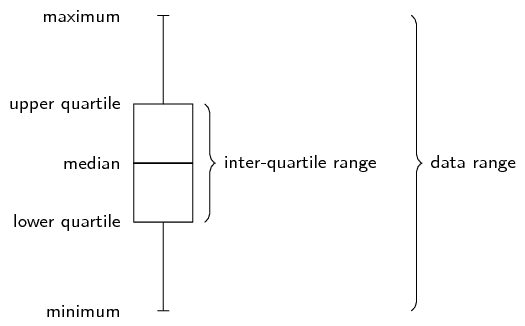## Worked example 3: Five number summary

Draw a box and whisker diagram for the following data set: $\text{1,25}\ ;\ \text{1,5}\ ;\ \text{2,5}\ ;\ \text{2,5}\ ;\ \text{3,1}\ ;\ \text{3,2}\ ;\ \text{4,1}\ ;\ \text{4,25}\ ;\ \text{4,75}\ ;\ \text{4,8}\ ;\ \text{4,95}\ ;\ \text{5,1}$

### Determine the minimum and maximum

Since the data set is already ordered, we can read off the minimum as the first value ($$\text{1,25}$$) and the maximum as the last value ($$\text{5,1}$$).

### Determine the quartiles

There are $$\text{12}$$ values in the data set.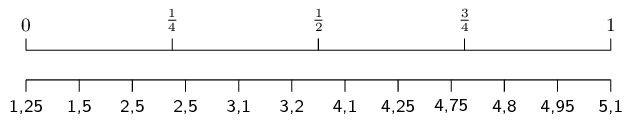Using the figure above we can see that the median is between the sixth and seventh values, making it. $\frac{\text{3,2}+\text{4,1}}{2} = \text{3,65}$

The first quartile lies between the third and fourth values, making it $Q_1 = \frac{\text{2,5}+\text{2,5}}{2} = \text{2,5}$

The third quartile lies between the ninth and tenth values, making it $Q_3 = \frac{\text{4,75}+\text{4,8}}{2} = \text{4,775}$

### Draw the box and whisker diagram

We now have the five number summary as ($$\text{1,25}$$; $$\text{2,5}$$; $$\text{3,65}$$; $$\text{4,775}$$; $$\text{5,1}$$). The box and whisker diagram representing the five number summary is given below.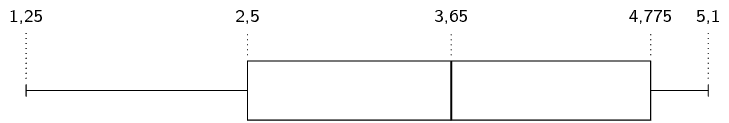## Revision

Textbook Exercise 11.1

For each of the following data sets, compute the mean and all the quartiles. Round your answers to one decimal place.

$$-\text{3,4}$$ ; $$-\text{3,1}$$ ; $$-\text{6,1}$$ ; $$-\text{1,5}$$ ; $$-\text{7,8}$$ ; $$-\text{3,4}$$ ; $$-\text{2,7}$$ ; $$-\text{6,2}$$

Mean: \begin{align*} \overline{x} &= \frac{(-\text{3,4}) + (-\text{3,1}) + (-\text{6,1}) + (-\text{1,5}) + (-\text{7,8}) + (-\text{3,4}) + (-\text{2,7}) + (-\text{6,2})}{8} \\ &\approx -\text{4,3} \end{align*}

To compute the quartiles, we order the data:

$$-\text{7,8}$$ ; $$-\text{6,2}$$ ; $$-\text{6,1}$$ ; $$-\text{3,4}$$ ; $$-\text{3,4}$$ ; $$-\text{3,1}$$ ; $$-\text{2,7}$$ ; $$-\text{1,5}$$

We use the diagram below to find at or between which values the quartiles lie.For the first quartile the position is between the second and third values. The second value is $$-\text{6,2}$$ and the third value is $$-\text{6,1}$$, which means that the first quartile is $$\frac{-\text{6,2} - \text{6,1}}{2}=-\text{6,15}$$.

For the median (second quartile) the position is halfway between the fourth and fifth values. Since both these values are $$-\text{3,4}$$, the median is $$-\text{3,4}$$.

For the third quartile the position is between the sixth and seventh values. Therefore the third quartile is $$-\text{2,9}$$.

$$-\text{6}$$ ; $$-\text{99}$$ ; $$\text{90}$$ ; $$\text{81}$$ ; $$\text{13}$$ ; $$-\text{85}$$ ; $$-\text{60}$$ ; $$\text{65}$$ ; $$-\text{49}$$

Mean: \begin{align*} \overline{x} &= \frac{(-\text{6}) + (-\text{99}) + (\text{90}) + (\text{81}) + (\text{13}) + (-\text{85}) + (-\text{60}) + (\text{65}) + (-\text{49})}{9} \\ &\approx -\text{5,6} \end{align*}

To compute the quartiles, we order the data:

$$-\text{99}$$ ; $$-\text{85}$$ ; $$-\text{60}$$ ; $$-\text{49}$$ ; $$-\text{6}$$ ; $$\text{13}$$ ; $$\text{65}$$ ; $$\text{81}$$ ; $$\text{90}$$

We use the diagram below to find at or between which values the quartiles lie.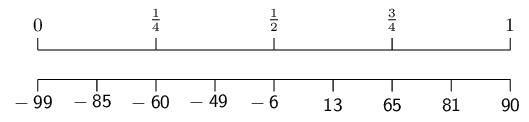We see that the quartiles are at $$-\text{60}$$; $$-\text{6}$$; and $$\text{65}$$.

$$\text{7}$$ ; $$\text{45}$$ ; $$\text{11}$$ ; $$\text{3}$$ ; $$\text{9}$$ ; $$\text{35}$$ ; $$\text{31}$$ ; $$\text{7}$$ ; $$\text{16}$$ ; $$\text{40}$$ ; $$\text{12}$$ ; $$\text{6}$$

The mean is $$\overline{x} = \text{18,5}$$.

To compute the quartiles, we order the data:

$3\ ;\ 6\ ;\ 7\ ;\ 7\ ;\ 9\ ;\ 11\ ;\ 12\ ;\ 16\ ;\ 31\ ;\ 35\ ;\ 40\ ;\ 45$

We use the diagram below to find at or between which values the quartiles lie.For the first quartile the position is between the third and fourth values. Since both these values are equal to $$\text{7}$$, the first quartile is $$\text{7}$$.

For the median (second quartile) the position is halfway between the sixth and seventh values. The sixth value is $$\text{11}$$ and the seventh value is $$\text{12}$$, which means that the median is $$\frac{11+12}{2}=\text{11,5}$$.

For the third quartile the position is between the ninth and tenth values. Therefore the third quartile is $$\frac{31+35}{2}=33$$.

Use the following box and whisker diagram to determine the range and inter-quartile range of the data.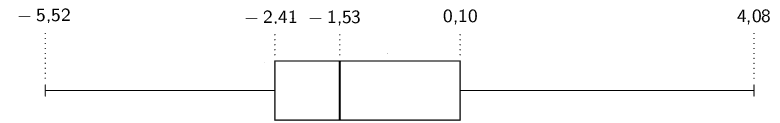The range is the difference between the minimum and maximum values. From the box-and-whisker diagram, the minimum is $$-\text{5,52}$$ and the maximum is $$\text{4,08}$$. Therefore the range is $$\text{4,08} - (-\text{5,52}) = \text{9,6}$$.

The inter-quartile range is the difference between the first and third quartiles. From the box-and-whisker diagram, the first quartile is $$-\text{2,41}$$ and the third quartile is $$\text{0,10}$$. Therefore the inter-quartile is $$\text{0,10} - (-\text{2,41}) = \text{2,51}$$.

Draw the box and whisker diagram for the following data.

$\text{0,2}\ ;\ -\text{0,2}\ ;\ -\text{2,7}\ ;\ \text{2,9}\ ;\ -\text{0,2}\ ;\ -\text{4,2}\ ;\ -\text{1,8}\ ;\ \text{0,4}\ ;\ -\text{1,7}\ ;\ -\text{2,5}\ ;\ \text{2,7}\ ;\ \text{0,8}\ ;\ -\text{0,5}$